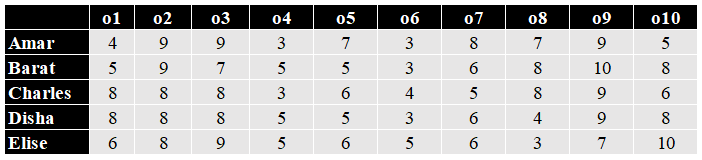### CAT 2021 Question Paper (Slot 2) Question 34

Instructions

Ten objects o1, o2, …, o10 were distributed among Amar, Barat, Charles, Disha, and Elise. Each item went to exactly one person. Each person got exactly two of the items, and this pair of objects is called her/his bundle.

The following table shows how each person values each object.The value of any bundle by a person is the sum of that person’s values of the objects in that bundle. A person X envies another person Y if X values Y’s bundle more than X’s own bundle.

For example, hypothetically suppose Amar’s bundle consists of o1 and o2, and Barat’s bundle consists of o3 and o4. Then Amar values his own bundle at 4 + 9 = 13 and Barat’s bundle at 9 + 3 = 12. Hence Amar does not envy Barat. On the other hand, Barat values his own bundle at 7 + 5 = 12 and Amar’s bundle at 5 + 9 = 14. Hence Barat envies Amar.

The following facts are known about the actual distribution of the objects among the five people.
1. If someone’s value for an object is 10, then she/he received that object.
2. Objects o1, o2, and o3 were given to three different people.
3. Objects o1 and o8 were given to different people.
4. Three people value their own bundles at 16. No one values her/his own bundle at a number higher than 16.
5. Disha values her own bundle at an odd number. All others value their own bundles at an even number.
6. Some people who value their own bundles less than 16 envy some other people who value their own bundle at 16. No one else envies others.

Question 34

# What BEST can be said about the distribution of object o1?

Solution

We have the following table :o10 is given to Elise and o9 is given to Bharat .
Now as Elise values his own bundle at an even number so the only two objects which can be given to Elise is o1 or o5 or o7.
Case 1 :
o1 is given to Elise
Now the total valuation of Elise = 12
Valuation of Disha is an odd number
So we can say Amar , Bharat and Charles values their bundles at 16 .
So for Bharat the valuation to be 16, o7 will be given to him
so we get
Bharat - o9 and o7 and Elise -o10 and o1
For charles to have valuation 16
the only way = 8+8
so we can say o8 is given to charles along with either o2 or o3 .(o1 and o8 cannot be together )
Now for Amart to have a valuation of 16
the only way possible = 9+7
Now so we can say
Amar will receive either o2 or o3 and o5 .
Now we are left with 04 and o6
So if Disha receives o4 and o6
The valuation of Disha will be 5+3 =8 which is not an odd number

Case 2 Elise receives o5 or o7 .
Now Valuation of Elise = 16 .
And Elise receives o10 and o5/o7.
Bharat received o9 and we know the evaluation of Bharat is an even number and the minimum even number possible for valuation of Bharat is 16 and no one can have evaluation more than 16 so Bharat received o7 .
So we have
Bharat - o9 ,o7
Elise -o10,o5.
Now as we know o1 ,o2 and o3 are given to three different persons so they are Amar, Charles and Disha .
Now As per Amar
he values Bharat at 17 so he envy him
So Amar will value his bundle less than 16
So the only possibility for Amar to value his bundle less than 16 = 12 =9+3.
Now we can say Charu will have 16 as his own valuation so he will get 8+8 .
Now o8 will be given to Charu, and he cannot have o1 , also he cannot have o2 because if he has o2 he will value Bharat’s bundle as 17 and will envy him which is not possible so Charu will have o3,o8
Now Amar will have o2 and Disha will have o1.
Now Amar will not have o4 because in that case Charles will envy Amar and is not possible so we can say Amar will have o6 and Disha will have o4.
So we have the following :

Amar - o2,o6

Bharat -o9,o7

Charu -o3,o8
Disha o1,o4
Elise -o10,o5

So o1 is given to Disha